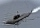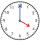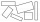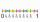# Fraction calculator

The calculator performs basic and advanced operations with fractions, expressions with fractions combined with integers, decimals, and mixed numbers. It also shows detailed step-by-step information about the fraction calculation procedure. Solve problems with two, three, or more fractions and numbers in one expression.

## Result:

### 22/3 + 31/12 = 23/4 = 5 3/4 = 5.75

Spelled result in words is twenty-three quarters (or five and three quarters).

### How do you solve fractions step by step?

1. Conversion a mixed number 2 2/3 to a improper fraction: 2 2/3 = 2 2/3 = 2 · 3 + 2/3 = 6 + 2/3 = 8/3

To find a new numerator:
a) Multiply the whole number 2 by the denominator 3. Whole number 2 equally 2 * 3/3 = 6/3
b) Add the answer from previous step 6 to the numerator 2. New numerator is 6 + 2 = 8
c) Write a previous answer (new numerator 8) over the denominator 3.

Two and two thirds is eight thirds
2. Conversion a mixed number 3 1/12 to a improper fraction: 3 1/12 = 3 1/12 = 3 · 12 + 1/12 = 36 + 1/12 = 37/12

To find a new numerator:
a) Multiply the whole number 3 by the denominator 12. Whole number 3 equally 3 * 12/12 = 36/12
b) Add the answer from previous step 36 to the numerator 1. New numerator is 36 + 1 = 37
c) Write a previous answer (new numerator 37) over the denominator 12.

Three and one twelfth is thirty-seven twelfths
3. Add: 8/3 + 37/12 = 8 · 4/3 · 4 + 37/12 = 32/12 + 37/12 = 32 + 37/12 = 69/12 = 3 · 23/3 · 4 = 23/4
For adding, subtracting, and comparing fractions, it is suitable to adjust both fractions to a common (equal, identical) denominator. The common denominator you can calculate as the least common multiple of both denominators - LCM(3, 12) = 12. In practice, it is enough to find the common denominator (not necessarily the lowest) by multiplying the denominators: 3 × 12 = 36. In the following intermediate step, cancel by a common factor of 3 gives 23/4.
In other words - eight thirds plus thirty-seven twelfths = twenty-three quarters.

#### Rules for expressions with fractions:

Fractions - simply use a forward slash between the numerator and denominator, i.e., for five-hundredths, enter 5/100. If you are using mixed numbers, be sure to leave a single space between the whole and fraction part.
The slash separates the numerator (number above a fraction line) and denominator (number below).

Mixed numerals (mixed fractions or mixed numbers) write as integer separated by one space and fraction i.e., 1 2/3 (having the same sign). An example of a negative mixed fraction: -5 1/2.
Because slash is both signs for fraction line and division, we recommended use colon (:) as the operator of division fractions i.e., 1/2 : 3.

Decimals (decimal numbers) enter with a decimal point . and they are automatically converted to fractions - i.e. 1.45.

The colon : and slash / is the symbol of division. Can be used to divide mixed numbers 1 2/3 : 4 3/8 or can be used for write complex fractions i.e. 1/2 : 1/3.
An asterisk * or × is the symbol for multiplication.
Plus + is addition, minus sign - is subtraction and ()[] is mathematical parentheses.
The exponentiation/power symbol is ^ - for example: (7/8-4/5)^2 = (7/8-4/5)2

#### Examples:

subtracting fractions: 2/3 - 1/2
multiplying fractions: 7/8 * 3/9
dividing Fractions: 1/2 : 3/4
exponentiation of fraction: 3/5^3
fractional exponents: 16 ^ 1/2
adding fractions and mixed numbers: 8/5 + 6 2/7
dividing integer and fraction: 5 ÷ 1/2
complex fractions: 5/8 : 2 2/3
decimal to fraction: 0.625
Fraction to Decimal: 1/4
Fraction to Percent: 1/8 %
comparing fractions: 1/4 2/3
multiplying a fraction by a whole number: 6 * 3/4
square root of a fraction: sqrt(1/16)
reducing or simplifying the fraction (simplification) - dividing the numerator and denominator of a fraction by the same non-zero number - equivalent fraction: 4/22
expression with brackets: 1/3 * (1/2 - 3 3/8)
compound fraction: 3/4 of 5/7
fractions multiple: 2/3 of 3/5
divide to find the quotient: 3/5 ÷ 2/3

The calculator follows well-known rules for order of operations. The most common mnemonics for remembering this order of operations are:
PEMDAS - Parentheses, Exponents, Multiplication, Division, Addition, Subtraction.
BEDMAS - Brackets, Exponents, Division, Multiplication, Addition, Subtraction
BODMAS - Brackets, Of or Order, Division, Multiplication, Addition, Subtraction.
GEMDAS - Grouping Symbols - brackets (){}, Exponents, Multiplication, Division, Addition, Subtraction.
Be careful, always do multiplication and division before addition and subtraction. Some operators (+ and -) and (* and /) has the same priority and then must evaluate from left to right.

## Fractions in word problems:

• An orchardDuring a visit to an orchard, Greg picked 3/5 of a bag of delicious golden apples, 4/5 of a bag of Macintosh apples, 2/5 of a bag of Cortland apples, 1/5 of a bag of Bartlett pears, and 4/5 of a bag of Bosch pears. How many bags of fruit to Greg pick in t
• Circular gardenAlice creates a circular vegetable garden. Tomatoes are planted in 1/3 of the circular garden, carrots are planted in 2/5 of the circular garden, and green peppers are planted in 1/10 of the circular garden. What fraction represents the remaining unplante
• Weigh in totalI put 3/5 kg of grapes into a box which is 1/4kg in weight. How many kilograms do the grapes and the box weigh in total?
• Honey 3Honey jogged 1 3/4 km on Monday,1 1/2 km on Wednesday and 1  2/3 km on Friday . how far did he jog?
• Math testBrayden was solving some math problems for the math team. He answered 2 math problems. Matthew answered 3, John answered 1 reasoning. Matthew 1/2 times as many. Brayden said that 2/6. Is he correct? Why or why not? Be sure to explain your answer.
• Evaluate 17Evaluate 2x+6y when x=- 4/5 and y=1/3. Write your answer as a fraction or mixed number in simplest form.
• SubmergingMonika dove 9 meters below the ocean's surface. She then dove 13 meters deeper. Then she rose 19 and one-fourth meters. What was her position concerning the water's surface (the water surface = 0, minus values = above water level, plus = above water level
• Flowers 4A flower seller has 4.05 kg of orange flowers and 6.50 kg of red flowers. He made flower baskets with 2.11 kg of both red and orange flowers in each. How many baskets did he make?
• Bus vs trainMilada took the bus and the journey took 55 minutes. Jarmila was 1h 20 min by train. They arrived in Prague at the same time 10h45 min. At what time did each have to go out?
• Fractions and mixed numerals(a) Convert the following mixed numbers to improper fractions. i. 3 5/8 ii. 7 7/6 (b) Convert the following improper fraction to a mixed number. i. 13/4 ii. 78/5 (c) Simplify these fractions to their lowest terms. i. 36/42 ii. 27/45 2. evaluate the follow
• Three rectanglesSome wire is used to make 3 rectangles: A, B, and C. Rectangle B's dimensions are 3/5 cm larger than Rectangle A's dimensions, and Rectangle C's dimensions are again 3/5 cm larger than Rectangle B's dimensions. Rectangle A is 2 cm by 3 1/5 cm. What is the
• StockEnterprise sold 7/12 of their products to foreign markets and 2/5 of the remainder sold at home. How many % of the products is still in stock?Max is working out 2/3+7/9. He says the answer is 9/12. What mistake have Max made?